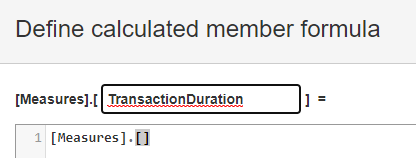# Time Dimension - how to create a calculated measure with the date?

Dear all,

let’s say we have a simple data sets of transactions, with attributes like

number, transaction_creation_date, transaction_end_date

(SQL import into private eazyBI)

As described here, there is only one time dimension (rule #2):
https://docs.eazybi.com/eazybi/data-import/data-mapping

So transactions_creation_date will become the time dimension - that works fine.

end_date would be configured also according to the documentation, as described under rule #4
(there it is called transaction cancel date).
Also works fine during the import.

My question :
How can I create a calculated measure when creating a report, that calculates for example “Transaction Duration” … so end_date - creation_date ?

My first problem is already in the dialogue, where I do not know how I can just use the creation date for calculation …There is no measure for the creation date, when importing like described above.I hope the question is understandable and big thank you in advance.

Best regards,
Markus

Hi @markusd!

You are on the right track with the end date! You should import the start date in the same way - both as property and Time count measure. Then you would have two measures grouped on Time dimension by the date and also properties for each Transaction to do the calculation with DateDiffDays(…) function.

Here is the mapping example:

Lauma / support@eazybi.com

1 Like

Hello lauma,

awesome thank you very much1 Like